#### 期刊菜单

Research Based on the Price Distribution of Clothing Single Category
DOI: 10.12677/WER.2022.111010, PDF, HTML, XML, 下载: 70  浏览: 110

Abstract: Apparel companies have a sales lead time, and they need to make sales plans in advance based on market demand. Based on the market niche theory and focusing on the retail clothing field, this paper analyzes the market segments formed by clothing single categories and the demand distri-bution based on the price dimension. Taking the sales data set of a retail clothing company as an example, we select the gamma distribution density function, the Logistic distribution function, and the normal distribution function to fit the demand distribution of the outerwear category. The re-sults show that, for the clothing single category market segment, the relationship between price and sales volume can be described by the gamma distribution density function. Based on this de-mand distribution, it can provide certain guidance for corporate sales planning.

1. 引言

2. 问题描述

2.1. 基于小生境理论的价格需求分布

2.1.1. 小生境理论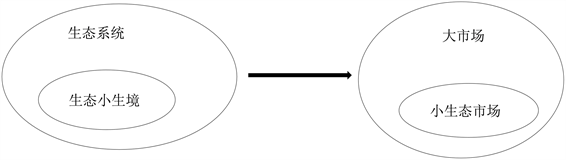Figure 1. Ecological niche and market niche

2.1.2. 聚焦于服装领域的小生境理论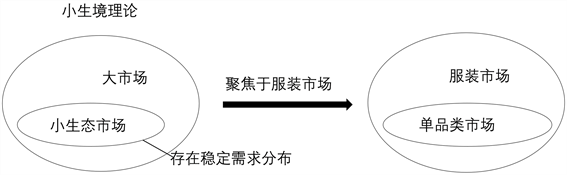Figure 2. Clothing category market based on niche theory

2.2. 数据特征分析

“人”、“货”、“场”作为零售市场三个核心要素，企业在日常经营中围绕三要素形成了销售数据集。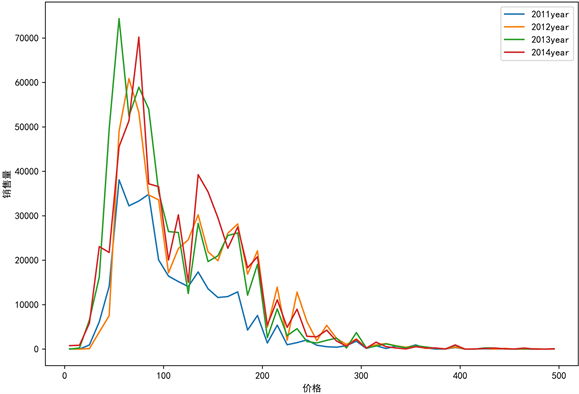Figure 3. Outerwear category price-sales relationship graph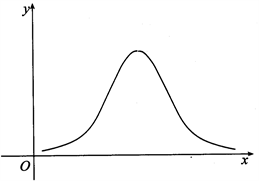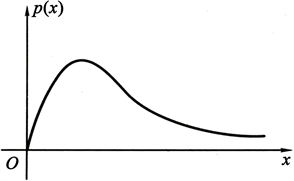Figure 4. Common distribution model curve

3. 需求分布模型选择

3.1. 常见的概率分布函数

1) 伽玛分布密度函数

$f\left(x,\alpha ,\beta \right)=\frac{{\beta }^{\alpha }}{\Gamma \left(\alpha \right)}{x}^{a-1}{\text{e}}^{-\beta x},x\ge 0$ (1)

$\Gamma \left(a\right)={\int }_{0}^{\infty }{t}^{a-1}{\text{e}}^{-t}\text{d}t$ (2)

2) Logistic分布密度函数

Logistic分布密度函数为：

$f\left(x\right)=\frac{{\text{e}}^{\frac{x-\mu }{\sigma }}}{\sigma {\left[1+{\text{e}}^{-\frac{x-\mu }{\sigma }}\right]}^{2}}$ (3)

Logistic分布函数是一种常见的S型函数，其取值介于0与1之间，分布函数指当n趋于无穷大时，从指数分布中抽取的容量n的随机样本的最大与最小样本值的平均的极限分布。分布函数常用于解决二分类问题的方法，而其密度函数曲线与伽玛及正态分布曲线相似，在相关文献中被作为参数模型进行需求分布拟合，其中， $\mu$ 表示位置参数， $\sigma$ 表示形状参数。

3) 正态分布密度函数

$g\left(x\right)=\frac{1}{\sigma \sqrt{2\pi }}{\text{e}}^{-\frac{-{\left(x-\mu \right)}^{2}}{2{\sigma }^{2}}}$ (4)

$x\in R$, $\mu ,\sigma$ 为常数，且 $\sigma >0$，称 $\epsilon$ 服从参数为 $\mu ,\sigma$ 的正态分布，用 $N\left(\mu ,{\sigma }^{2}\right)$ 表示， $f\left(x\right)$ 表示式可间记为 $N\left(\mu ,{\sigma }^{2}\right)$$N\left(\mu ,\sigma \right)$。正态分布曲线广泛存在于自然现象、社会现象以及科学技术及生产活动中，在实际中遇到的许多随机问题都服从或者近似服从正态分布，且近似分布经过一定转换之后，依然服从正态或者近似正态分布，正态分布是最重要的概率分布，在自然科学与社会科学中应用广泛 。

3.2. 参数估计方法

$l\left(\theta \right)=P\left(D|\theta \right)=p\left\{{x}_{1},{x}_{2},\cdots ,{x}_{n}|\theta \right\}=\underset{i=1}{\overset{n}{\prod }}P\left({x}_{i}|\theta \right)$

$\stackrel{^}{\theta }=\mathrm{arg}\mathrm{max}l\left(\theta \right)=\mathrm{arg}\mathrm{max}\underset{i=1}{\overset{n}{\prod }}P\left({x}_{i}|\theta \right)$

$\stackrel{^}{\theta }=\mathrm{arg}\mathrm{max}H\left(\theta \right)=\mathrm{arg}\mathrm{max}\mathrm{ln}l\left(\theta \right)=\mathrm{arg}\mathrm{max}\mathrm{ln}\underset{i=1}{\overset{n}{\prod }}P\left({x}_{i}|\theta \right)=\mathrm{arg}\mathrm{max}\underset{i=1}{\overset{n}{\sum }}\mathrm{ln}pP\left({x}_{i}|\theta \right)$

4. 需求分布拟合Table 1. Parameter estimation result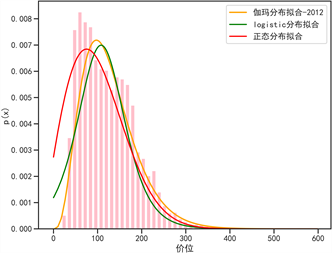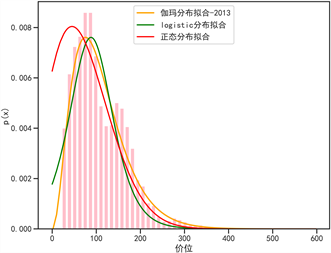Figure 5. Demand distribution fitting graph

$I=\sum {\left({y}_{i}-{{y}^{\prime }}_{i}\right)}^{2}$ (5)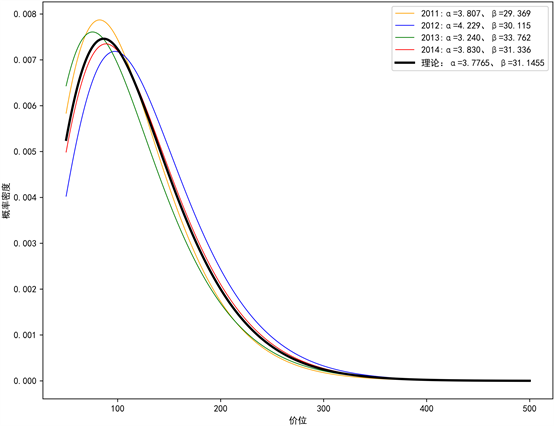Figure 6. Gamma distribution curve

5. 结论

  Odlin, D. and Benson-Rea, M. (2021) Market Niches as Dynamic, Co-Created Resource Domains. Industrial Marketing Management, 95, 29-40. https://doi.org/10.1016/j.indmarman.2021.03.008  Choi, T.-M., Hui, C.-L., Liu, N., et al. (2014) Fast Fashion Sales Forecasting with Limited Data and Time. Decision Support Systems, 59, 84-92. https://doi.org/10.1016/j.dss.2013.10.008  于国瑞. 服装流行要素识别与品牌服装设计[J]. 纺织学报, 2004(1): 126-128+6.  King, J.A. (2011) 9-Colour Trend Forecasting and Its Influence on the Fashion and Textile Industry. Textile Design, 193-206. https://doi.org/10.1533/9780857092564.3.193  赵雅彬, 朱伟明. 零售服装品牌线上线下渠道冲突与价格博弈研究[J]. 上海纺织科技, 2017, 45(10): 61-63.  Zhang, J., Onal, S. and Das, S. (2017) Price Differentiated Channel Switching in a Fixed Period Fast Fashion Supply Chain. International Journal of Production Economics, 193, 31-39. https://doi.org/10.1016/j.ijpe.2017.06.030  Ulrich, M., Jahnke, H., Langrock, R., et al. (2019) Distributional Regression for Demand Forecasting in e-Grocery. European Journal of Operational Research. https://doi.org/10.2139/ssrn.3312609  Momen, S. and Torabi, S.A. (2021) Om-ni-Channel Retailing: A Data-Driven Distributionally Robust Approach for Integrated Fulfillment Services under Compe-tition with Traditional and Online Retailers. Computers & Industrial Engineering, 157, 107353. https://doi.org/10.1016/j.cie.2021.107353  Afshar-Nadjafi, B., Mashatzadeghan, H. and Khamseh, A. (2016) Time-Dependent Demand and Utility-Sensitive Sale Price in a Retailing System. Journal of Retailing and Consumer Ser-vices, 32, 171-174. https://doi.org/10.1016/j.jretconser.2016.06.011  李琪菡, 张德银, 罗英, 等. 地铁功率波动的概率分布特性分析[J]. 电测与仪表, 2020, 57(3): 26-31+45.  李德毅, 刘常昱. 论正态云模型的普适性[J]. 中国工程科学, 2004, 6(8): 28-34.